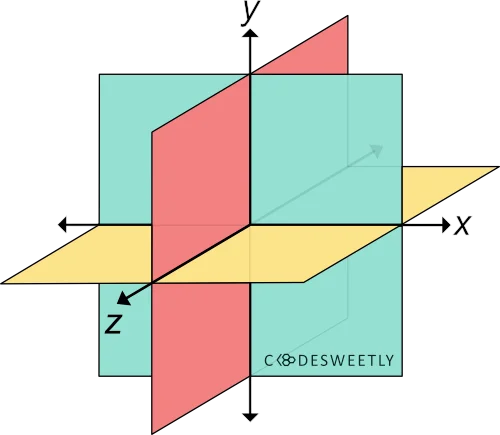# CSS rotateZ() Function – How to Rotate Elements around Z-axis

rotateZ() transforms an element by rotating it three-dimensionally around the Z-axis.A three-dimensional Cartesian coordinate system showing the X-, Y-, and Z-axis

## Syntax of the CSS rotateZ() Function​

rotateZ() accepts a single argument. Here is the syntax:

element {  transform: rotateZ(angle);}

Note the following:

## Examples of the CSS rotateZ() Function​

Below are some examples of how the CSS rotateZ() function works.

### How to do a zero-degree rotation around the Z-axis​

img {  transform: rotateZ(0deg);  width: 80%;}

Try Editing It

The snippet above used the rotateZ() function to specify a zero-degree (0⁰) rotation for the image around the Z-axis.

### How to do a 70-degree rotation around the Z-axis​

img {  transform: rotateZ(70deg);  width: 80%;}

Try Editing It

The snippet above used the rotateZ() function to specify a seventy-degree (70⁰) rotation for the image around the Z-axis.

## CSS rotateZ() Function vs. rotate Property: What's the Difference?​

CSS rotateZ() functions and CSS rotate property provide two similar ways to specify rotation transformations.

The main differences between the two rotation techniques are as follows:

• The CSS rotate property allows rotating an element without using the CSS transform property.
• The CSS rotate property's syntax is shorter than its function alternative.
• The CSS rotate property saves you from remembering the specific order to position the transform functions.
• Browsers calculate the transform functions' matrix in the order you assigned them to the CSS transform property—from left to right.
• Browsers calculate the transform properties' matrix in the following transformation matrix order:
1. translate
2. rotate
3. scale

Here's an example:

Use CSS rotate property to do a 70-degree rotation around the Z-axis:

img {  rotate: z 70deg; /* Equal to a transform: rotateZ(70deg) property */  width: 80%;}

Try Editing It

The snippet above used the rotate property to specify a seventy-degree (70⁰) rotation for the image around the Z-axis.

note

A none value tells browsers not to rotate the selected element.

## Overview​

This article discussed what a CSS rotateZ() function is. We also used examples to see how it works.